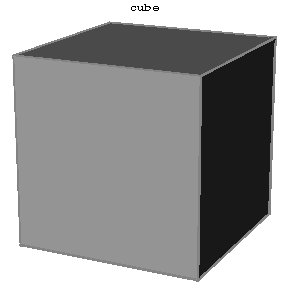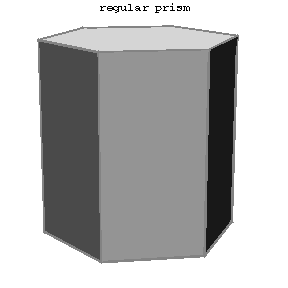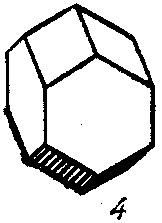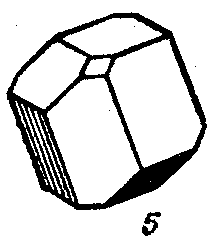# Parallelohedron

A polyhedron for which it is possible, using a parallel displacement, to fill the entire space in such a way that no two copies of the polyhedron intersect (except for their boundary faces) and such that there are no empty spaces left between them, i.e. they should form a partition of space. Examples of parallelohedra are a cube or a regular $6$-angled prism. Topologically, the set of edges of parallelohedra fall into five different lattice types (see Fig. a, Fig. b, Fig. c, Fig. d, Fig. e). The numbers of faces are 6, 8, 12, 12, 14. In order that a polyhedron is a parallelohedron it is necessary and sufficient that it is a convex polyhedron of one of the five indicated topological types and that all of its faces have centres of symmetry. The centres of the parallelohedra in a partition form a lattice of points (see Voronoi lattice types).Figure: p071440aFigure: p071440bFigure: p071440cFigure: p071440dFigure: p071440e